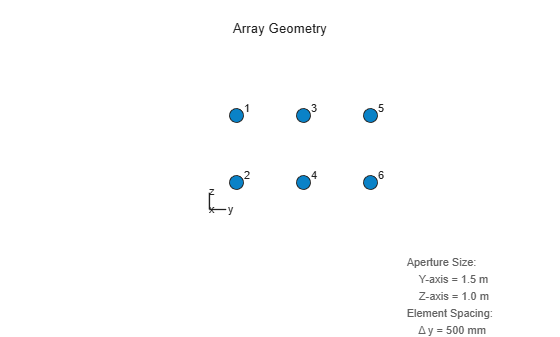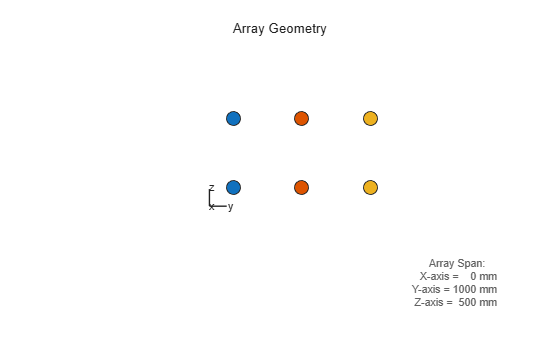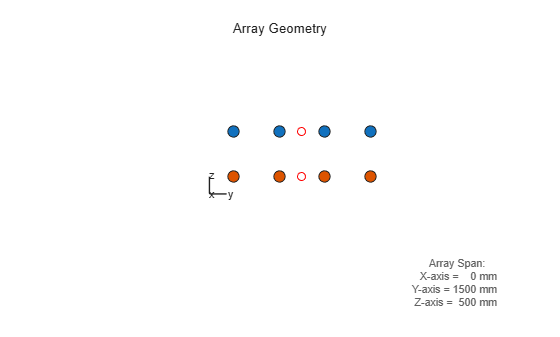Documentation

## Subarrays Within Arrays

### Definition of Subarrays

In Phased Array System Toolbox™ software, a subarray is an accessible subset of array elements. When you use an array that contains subarrays, you can access measurements from the subarrays but not from the individual elements. Similarly, you can perform processing at the subarray level but not at the level of the individual elements. As a result, the system has fewer degrees of freedom than if you controlled the system at the level of the individual elements.

### Benefits of Using Subarrays

Radar applications often use subarrays because operations, such as phase shifting and analog-to-digital conversion, are too expensive to implement for each element. It is less expensive to group the elements of an array through hardware, thus creating subarrays within the array. Grouping elements through hardware limits access to measurements and processing to the subarray level.

### Support for Subarrays Within Arrays

To work with subarrays, you must define the array and the subarrays within it. You can either define the array first or begin with the subarray. Choose one of these approaches:

• Define one subarray, and then build a larger array by arranging copies of the subarray. The subarray can be a ULA, URA, or conformal array. The copies are identical, except for their location and orientation. You can arrange the copies spatially in a grid or a custom layout.

When you use this approach, you build the large array by creating a `phased.ReplicatedSubarray` System object™. This object stores information about the subarray and how the copies of it are arranged to form the larger array.

• Define an array, and then partition it into subarrays. The array can be a ULA, URA, or conformal array. The subarrays do not need to be identical. A given array element can be in more than one subarray, leading to overlapped subarrays.

When you use this approach, you partition your array by creating a `phased.PartitionedArray` System object. This object stores information about the array and its subarray structure.

After you create a `phased.ReplicatedSubarray` or `phased.PartitionedArray` object, you can use it to perform beamforming, steering, or other operations. To do so, specify your object as the value of the `SensorArray` or `Sensor` property in objects that have such a property and that support subarrays. Objects that support subarrays in their `SensorArray` or `Sensor` property include:

• `phased.AngleDopplerResponse`

• `phased.ArrayGain`

• `phased.ArrayResponse`

• `phased.Collector`

• `phased.ConstantGammaClutter`

• `phased.MVDRBeamformer`

• `phased.PhaseShiftBeamformer`

• `phased.Radiator`

• `phased.STAPSMIBeamformer`

• `phased.SteeringVector`

• `phased.SubbandPhaseShiftBeamformer`

• `phased.WidebandCollector`

### Rectangular Array Partitioned into Linear Subarrays

This example shows how to set up a rectangular array containing linear subarrays. The example also finds the phase centers of the subarrays.

Create a 2-by-3 rectangular array.

`array = phased.URA('Size',[2 3]);`

Plot the positions of the array elements in the yz-plane (all x-coordinates are zero.) Include labels that indicate the numbering of the elements. The numbering is important for selecting which elements are included in each subarray.

`viewArray(array,'ShowIndex','All')`Create and view an array consisting of three 2-element linear subarrays each parallel to the z-axis. Use the indices from the plot to form the matrix for the `SubarraySelection` property. The `getSubarrayPosition` method returns the phase centers of the three subarrays.

```subarray1 = [1 1 0 0 0 0; 0 0 1 1 0 0; 0 0 0 0 1 1]; partitionedarray1 = phased.PartitionedArray('Array',array,... 'SubarraySelection',subarray1); viewArray(partitionedarray1)````subarray1pos = getSubarrayPosition(partitionedarray1)`
```subarray1pos = 3×3 0 0 0 -0.5000 0 0.5000 0 0 0 ```

Create and view another array consisting of two 3-element linear subarrays parallel to the y-axis. Using the `getSubarrayPosition` method, find the phase centers of the two subarrays.

```subarray2 = [0 1 0 1 0 1; 1 0 1 0 1 0]; partitionedarray2 = phased.PartitionedArray('Array',array,... 'SubarraySelection',subarray2); viewArray(partitionedarray2)````subarraypos2 = getSubarrayPosition(partitionedarray2)`
```subarraypos2 = 3×2 0 0 0 0 -0.2500 0.2500 ```

### Linear Subarray Replicated to Form Rectangular Array

This example shows how to arrange copies of a linear subarray to form a rectangular array.

Create a 4-element linear array parallel to the y-axis.

`array = phased.ULA('NumElements',4);`

Create a rectangular array by arranging two copies of the linear array.

`replsubarray = phased.ReplicatedSubarray('Subarray',array,'GridSize',[2 1]);`

Plot the positions of the array elements (filled circles) and the phase centers of the subarrays (unfilled circles). The elements lie in the yz-plane because all the x-coordinates are zero.

```viewArray(replsubarray); hold on; subarraypos = getSubarrayPosition(replsubarray); sx = subarraypos(1,:); sy = subarraypos(2,:); sz = subarraypos(3,:); scatter3(sx,sy,sz,'ro') hold off```### Linear Subarray Replicated in a Custom Grid

This example shows how to arrange copies of a linear subarray in a triangular layout.

Create a 4-element linear array.

```antenna = phased.CosineAntennaElement('CosinePower',1); array = phased.ULA('NumElements',4,'Element',antenna);```

Create a larger array by arranging three copies of the linear array. Define the phase centers and normal directions of the three copies explicitly.

```vertex_ang = [60 180 -60]; vertex = 2*[cosd(vertex_ang); sind(vertex_ang); zeros(1,3)]; subarray_pos = 1/2*[... (vertex(:,1)+vertex(:,2)) ... (vertex(:,2)+vertex(:,3)) ... (vertex(:,3)+vertex(:,1))]; replsubarray = phased.ReplicatedSubarray('Subarray',array,... 'Layout','Custom',... 'SubarrayPosition',subarray_pos,... 'SubarrayNormal',[120 0;-120 0;0 0].');```

Plot the positions of the array elements (blue) and the phase centers (red) of the subarrays. The plot is in the xy-plane because all the z-coordinates are zero.

```viewArray(replsubarray,'ShowSubarray',[]) hold on scatter3(subarray_pos(1,:),subarray_pos(2,:),... subarray_pos(3,:),'ro') hold off```